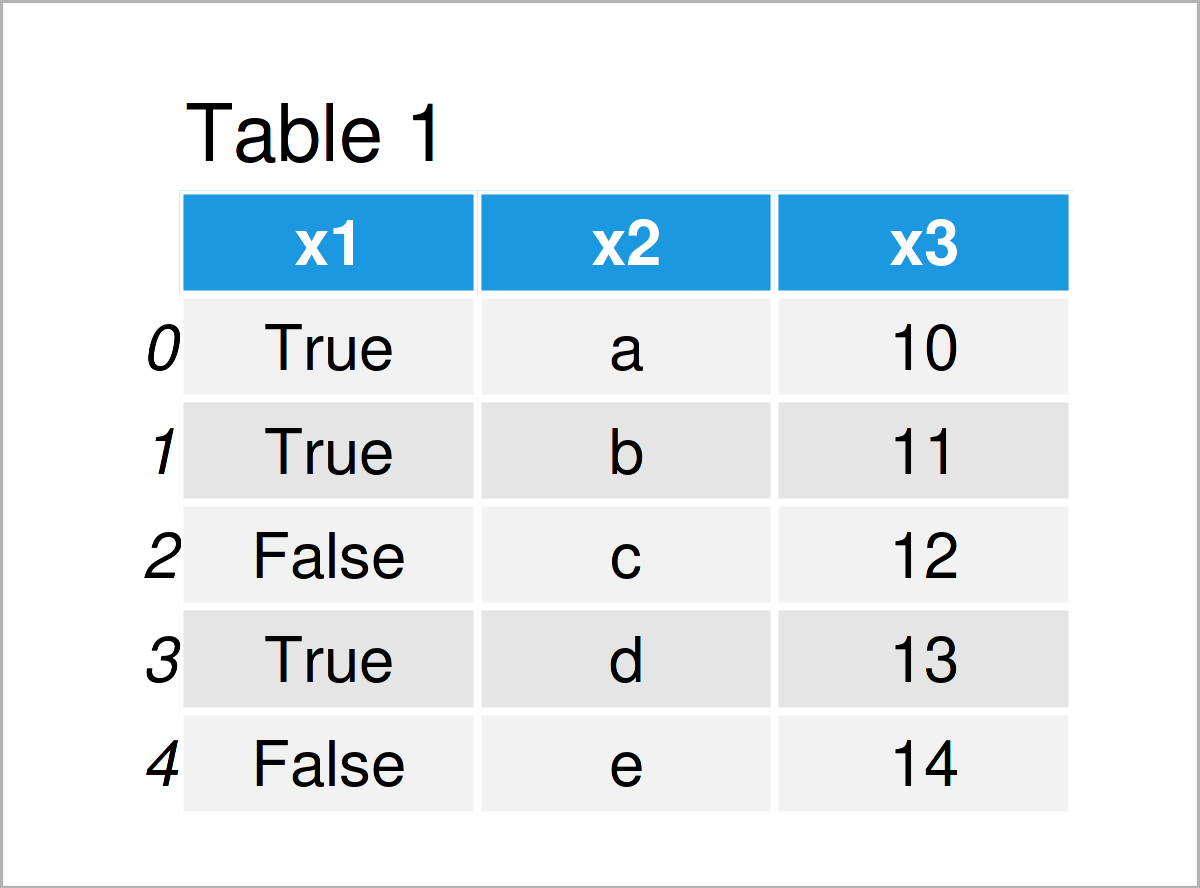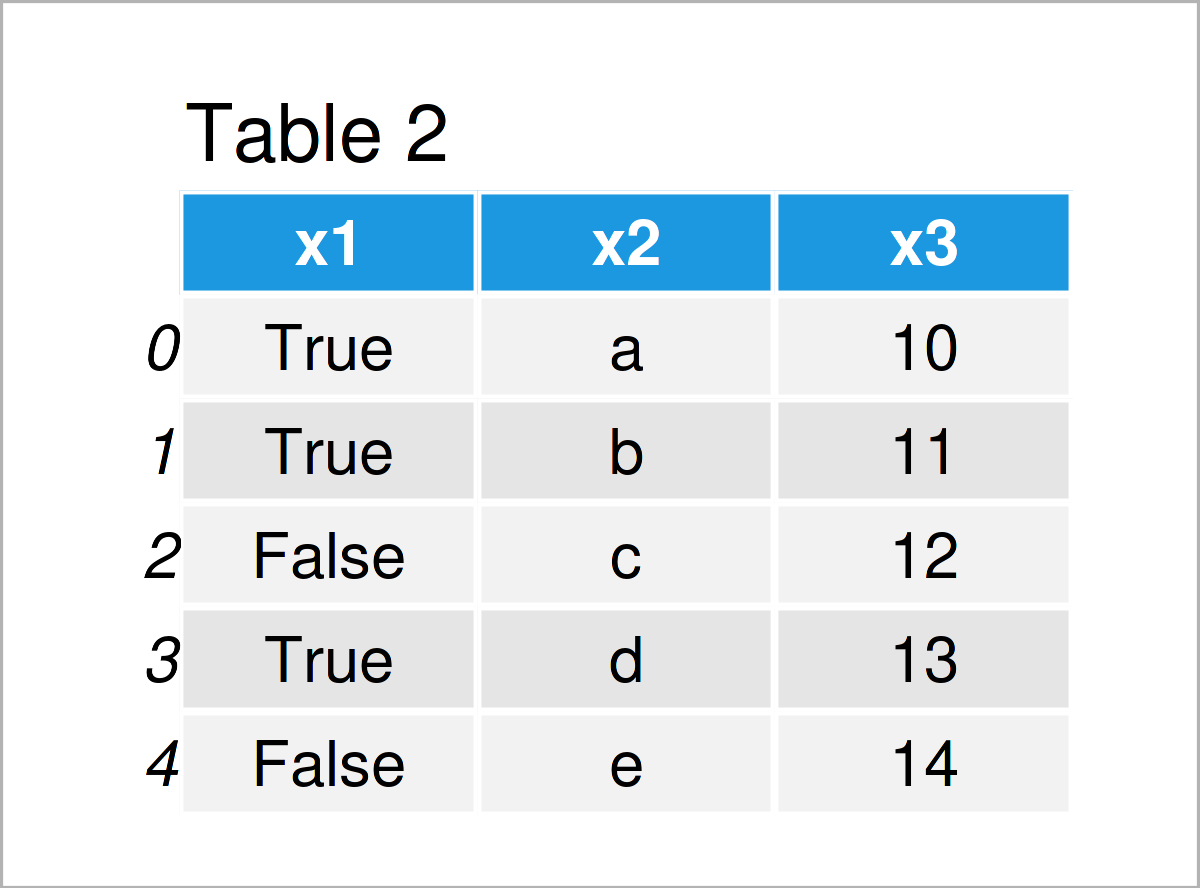# Convert True/False Boolean to String in pandas DataFrame Column in Python (2 Examples)

In this article, I’ll illustrate how to convert a True/False boolean column to the string data type in a pandas DataFrame in Python.

The tutorial contains the following contents:

Let’s take a look at some Python codes in action.

## Example Data & Add-On Libraries

In order to use the functions of the pandas library, we first have to import pandas:

`import pandas as pd                                                    # Import pandas library to Python`

As a next step, we’ll also have to define a pandas DataFrame that we can use in the examples later on:

```data = pd.DataFrame({'x1':[True, True, False, True, False],            # Create pandas DataFrame
'x2':['a', 'b', 'c', 'd', 'e'],
'x3':range(10, 15)})
print(data)                                                            # Print pandas DataFrame```Table 1 shows that our example data has five lines and three variables. The first column x1 contains a True/False boolean indicator.

## Example 1: Convert Boolean Data Type to String in Column of pandas DataFrame

In Example 1, I’ll demonstrate how to transform a True/False logical indicator to the string data type.

For this task, we can use the map function as shown below:

```data_new1 = data.copy()                                                # Create copy of pandas DataFrame
data_new1['x1'] = data_new1['x1'].map({True: 'True', False: 'False'})  # Replace boolean by string
print(data_new1)                                                       # Print updated pandas DataFrame```By executing the previously shown syntax, we have managed to create Table 2, i.e. a new pandas DataFrame.

This DataFrame looks exactly the same as our input data set. However, there’s one major difference that gets obvious when we are checking the data types of the columns in our new pandas DataFrame:

```print(data_new1.dtypes)                                                # Check data types of columns
# x1    object
# x2    object
# x3     int64
# dtype: object```

As you can see, the first column x1 has the object dtype (note that pandas stores strings as objects). This shows that we have converted the boolean data type of our input data set to a character string object.

## Example 2: Replace Boolean by String in Column of pandas DataFrame

In the first example, we have kept the wording True/False in our updated string column.

This section demonstrates how to change a boolean True/False indicator to different words.

Once again, we can use the map function:

```data_new2 = data.copy()                                                # Create copy of pandas DataFrame
data_new2['x1'] = data_new2['x1'].map({True: 'yes', False: 'no'})      # Replace boolean by string
print(data_new2)                                                       # Print updated pandas DataFrame```After executing the previous Python code the pandas DataFrame shown in Table 3 has been created. As you can see, the True values of our input data set have been converted to the character string ‘yes’, and the False elements have been switched to the character string ‘no’.

The class of this column has also been adjusted:

```print(data_new1.dtypes)                                                # Check data types of columns
# x1    object
# x2    object
# x3     int64
# dtype: object```

## Video & Further Resources

Do you need further information on the Python programming code of this tutorial? Then you may want to watch the following video on my YouTube channel. In the video, I explain the content of this page:

Additionally, you might want to have a look at the other tutorials on my website.

This article has illustrated how to transform a boolean column to the string data type in a pandas DataFrame in the Python programming language. In case you have further questions, don’t hesitate to let me know in the comments section below.

Subscribe to the Statistics Globe Newsletter